# Types of Controllers | Proportional Integral and Derivative Controllers

Contents

## What is a Controller?

In control systems, a controller is a mechanism that seeks to minimize the difference between the actual value of a system (i.e. the process variable) and the desired value of the system (i.e. the setpoint). Controllers are a fundamental part of control engineering and used in all complex control systems.

Before we introduce you to various controllers in detail, it is essential to know the uses of controllers in the theory of control systems. The important uses of the controllers include:

1. Controllers improve the steady-state accuracy by decreasing the steady state error.
2. As the steady-state accuracy improves, the stability also improves.
3. Controllers also help in reducing the unwanted offsets produced by the system.
4. Controllers can control the maximum overshoot of the system.
5. Controllers can help in reducing the noise signals produced by the system.
6. Controllers can help to speed up the slow response of an overdamped system.

Different varieties of these controllers are codified within industrial automotive devices such as programmable logic controllers and SCADA systems. The various types of controllers are discussed in detail below.

## Types of Controllers

There are two main types of controllers: continuous controllers, and discontinuous controllers.

In discontinuous controllers, the manipulated variable changes between discrete values. Depending on how many different states the manipulated variable can assume, a distinction is made between two position, three position, and multi-position controllers.

Compared to continuous controllers, discontinuous controllers operate on very simple, switching final controlling elements.

The main feature of continuous controllers is that the controlled variable (also known as the manipulated variable) can have any value within the controller’s output range.

Now in the continuous controller theory, there are three basic modes on which the whole control action takes place, which are:

1. Proportional controllers.
2. Integral controllers.
3. Derivative controllers.

We use the combination of these modes to control our system such that the process variable is equal to the setpoint (or as close as we can get it). These three types of controllers can be combined into new controllers:

1. Proportional and integral controllers (PI Controller)
2. Proportional and derivative controllers (PD Controller)
3. Proportional integral derivative control (PID Controller)

Now we will discuss each of these control modes in detail below.

### Proportional Controllers

All controllers have a specific use case to which they are best suited. We cannot just insert any type of controller at any system and expect a good result – there are certain conditions that must be fulfilled. For a proportional controller, there are two conditions and these are written below:

1. The deviation should not be large; i.e. there should not be a large deviation between the input and output.
2. The deviation should not be sudden.

Now we are in a condition to discuss proportional controllers, as the name suggests in a proportional controller the output (also called the actuating signal) is directly proportional to the error signal. Now let us analyze the proportional controller mathematically. As we know in proportional controller output is directly proportional to the error signal, writing this mathematically we have,

Removing the sign of proportionality we have,

Where Kp is proportional constant also known as controller gain.

It is recommended that Kp should be kept greater than unity. If the value of Kp is greater than unity (>1), then it will amplify the error signal and thus the amplified error signal can be detected easily.

#### Advantages of Proportional Controller

Now let us discuss some advantages of the proportional controller.

1. The proportional controller helps in reducing the steady-state error, thus makes the system more stable.
2. The slow response of the overdamped system can be made faster with the help of these controllers.

#### Disadvantages of Proportional Controller

Now there are some serious disadvantages of these controllers and these are written as follows:

1. Due to the presence of these controllers, we get some offsets in the system.
2. Proportional controllers also increase the maximum overshoot of the system.

Now, we will explain the Proportional Controller (P-controller) with a unique example. With this example reader’s knowledge about ‘Stability’ and ‘Steady State Error’ will also enhance. Consider the feedback control system shown in Figure-1

‘K’ is called a proportional controller (also called error amplifier). Characteristics equation of this control system can be written as:

s3+3s2+2s+K=0

If the Routh-Hurwitz is applied in this characteristics equation, then the range of ‘K’ for the stability can be found as 0<K<6. (It implies that for the values K>6 system will be unstable; for the value of K=0, the system will be marginally stable).

Root locus of above control system is shown in Figure-2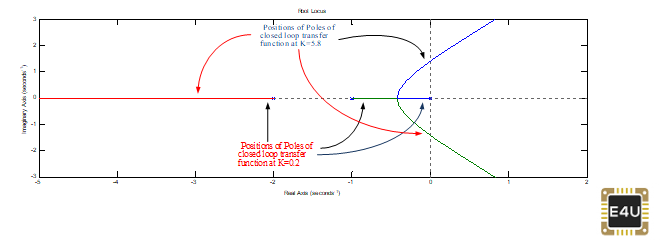Figure-2: Root locus of the system shown in Figure-1, Root Locus provides an idea that what should be the value of ‘K’

(You can understand that root locus is drawn for the open-loop transfer function (G(s)H(s), but it gives an idea about the poles of the closed-loop transfer function, i.e. roots of characteristics equation, also called zeros of the characteristics equation.

The Root locus is helpful in designing the value of ‘K’, i.e. gain of the proportional controller). So, the system (in Figure-1) is stable for values such as K= 0.2, 1, 5.8 etc.; but what value we should select. We will analyze each value and show you the results.

As a summary, you can understand that the high value of ‘K’ (i.e., for example, K=5.8) will reduce the stability (it is a disadvantage) but improves the steady-state performance (i.e. reduce the steady-state error, which will be an advantage).

You can understand that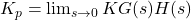, Steady state error (ess)=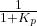(It is applicable in case of step input)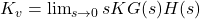, Steady state error (ess)=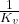(It is applicable in case of ramp input)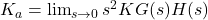, Steady state error (ess)=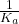(It is applicable in case of parabolic input)

It can be observed that for the high value of ‘K’, values of Kp, Kv and Ka will be high and steady-state error will below.

Now we will take each case and explain the results

1. At K=0.2

In this case characteristics equation of the system is s3+ 3s2+ 2s+0.2=0; roots of this equation are -2.088, -0.7909 and -0.1211; We can ignore -2.088 (as it is far away from imaginary axis). On the basis of the remaining two roots, it can be termed as an overdamped system (as both the roots are real & negative, no imaginary parts).

Against step input, its time response is shown in Fig-3. It can be seen that the response has no oscillations. (if roots are complex then time response exhibits oscillations). The overdamped system has damping more than ‘1’.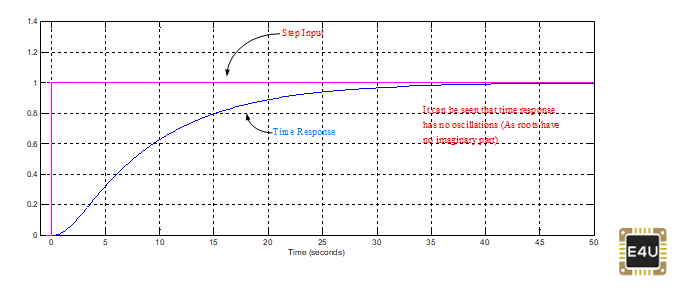Figure-3: Response has no oscillations, it is the response of the overdamped system

In the present case open loop transfer function is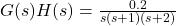Its Gain Margin (GM)=29.5 dB, Phase Margin (PM)=81.5°,

It should be noted that in the designing of control systems, overdamped systems are not preferred. Roots (poles of closed-loop transfer function) should have slight imaginary parts.

In the case of overdamped, damping is more than ‘1’, while damping around 0.8 is preferred.

2. At K=1

In this case characteristics equation of the system is s3+ 3s2+ 2s+1=0; roots of this equation are -2.3247, -0.3376 ±j0.5623; We can ignore -2.3247.

On the basis of the remaining two roots, it can be termed as an underdamped system (as both the roots are complex having negative real parts). Against step input, its time response is shown in Fig-4.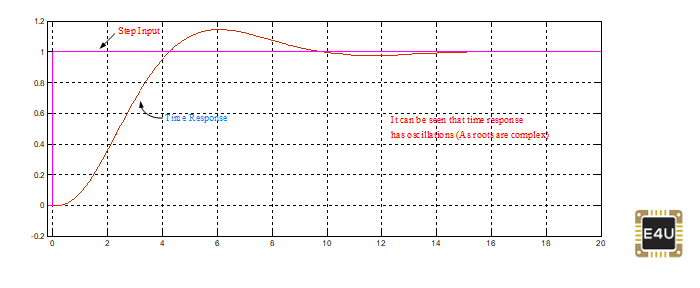Figure-4: Response has oscillations, it is the response of an underdamped system

In the present case open loop transfer function is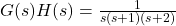Its Gain Margin (GM)=15.6 dB, Phase Margin (PM)=53.4°,

3. At K=5.8

As 5.8 is very close to 6, so you can understand that system is stable, but almost on the border. You can find the roots of its characteristics equation.

One root can be ignored, the remaining two roots will be very close to the imaginary axis. (Roots of its characteristics equation will be -2.9816, -0.0092±j1.39). Against step input, its time response is shown in Fig-5.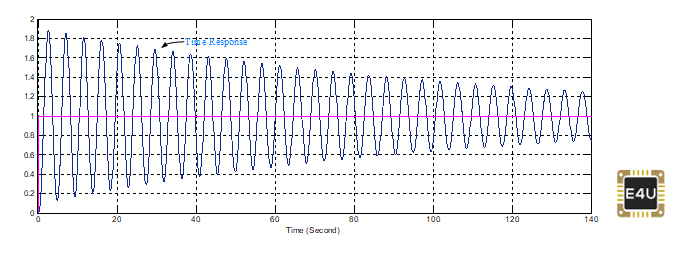Figure-5: Response has oscillations, it is the response of the underdamped system (Response in Figure-4 is also belongs to the underdamped system)

In the present case open loop transfer function is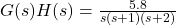Its Gain Margin=0.294 db, Phase Margin =0.919°

It can be analyzed, as compared to the previous cases, GM & PM are reduced drastically. As the system is very close to instability, hence GM & PM are also very close to zero value.

### Integral Controllers

As the name suggests in integral controllers the output (also called the actuating signal) is directly proportional to the integral of the error signal. Now let us analyze integral controller mathematically.

As we know in an integral controller output is directly proportional to the integration of the error signal, writing this mathematically we have,

Removing the sign of proportionality we have,

Where Ki is an integral constant also known as controller gain. The integral controller is also known as reset controller.

#### Advantages of Integral Controller

Due to their unique ability, Integral Controllers can return the controlled variable back to the exact set point following a disturbance that’s why these are known as reset controllers.

#### Disadvantages of Integral Controller

It tends to make the system unstable because it responds slowly towards the produced error.

### Derivative Controllers

We never use derivative controllers alone. It should be used in combinations with other modes of controllers because of its few disadvantages which are written below:

1. It never improves the steady-state error.
2. It produces saturation effects and also amplifies the noise signals produced in the system.

Now, as the name suggests in a derivative controller the output (also called the actuating signal) is directly proportional to the derivative of the error signal.

Now let us analyze the derivative controller mathematically. As we know in a derivative controller output is directly proportional to the derivative of the error signal, writing this mathematically we have,

Removing the sign of proportionality we have,

Where, Kd is proportional constant also known as controller gain. The derivative controller is also known as the rate controller.

#### Advantages of Derivative Controller

The major advantage of a derivative controller is that it improves the transient response of the system.

### Proportional and Integral Controller

As the name suggests it is a combination of proportional and an integral controller the output (also called the actuating signal) is equal to the summation of proportional and integral of the error signal.

Now let us analyze proportional and integral controller mathematically.

As we know in a proportional and integral controller output is directly proportional to the summation of proportional of error and integration of the error signal, writing this mathematically we have,

Removing the sign of proportionality we have,

Where, Ki and kp proportional constant and integral constant respectively.

Advantages and disadvantages are combinations of the advantages and disadvantages of proportional and integral controllers.

Through the PI controller, we are adding one pole at origin and one zero somewhere away from the origin (in the left-hand side of complex plane).

As the pole is at the origin, its effect will be more, hence PI controller may reduce the stability; but its main advantage is that it reduces steady-state error drastically, due for this reason it is one of the most widely used controllers.

The schematic diagram of the PI controller is shown in Fig-6. Against step input, For the values of K=5.8, Ki=0.2, Its time response, is shown in Fig-7. At K=5.8 (As a P- controller, it was on the verge of instability, so just by adding the small value of an Integral part, it became unstable.

Please note the Integral part reduces the stability, which does not mean that system will be always unstable. In the present case, we have added an integral part and the system became unstable).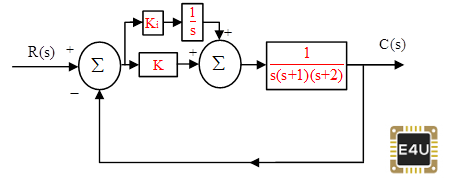Figure-6: The closed loop control system with PI Controller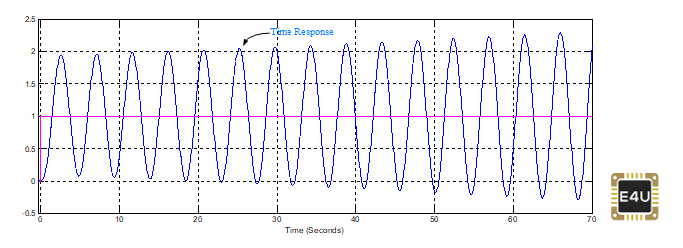Figure-7: The response of the system shown in Figure-6, with K=5.8, Ki=0.2

### Proportional and Derivative Controller

As the name suggests it is a combination of proportional and a derivative controller the output (also called the actuating signal) is equals to the summation of proportional and derivative of the error signal. Now let us analyze proportional and derivative controller mathematically.

As we know in a proportional and derivative controller output is directly proportional to the summation of proportional of error and differentiation of the error signal, writing this mathematically we have,

Removing the sign of proportionality we have,

Where, Kd and Kp proportional constant and derivative constant respectively.
Advantages and disadvantages are combinations of advantages and disadvantages of proportional and derivative controllers.

Readers should note that adding ‘zero’ at the proper location in the open-loop transfer function improves stability, while the addition of pole in the open-loop transfer function may reduce the stability.

The words “at proper location” in the above sentence are very important & it is called designing of the control system (i.e. both zero & pole should be added at proper points in the complex plane to get the desired result).

Inserting the PD controller is like the addition of zero in open-loop transfer function [G(s)H(s)]. Diagram of PD Controller is shown in Fig-8

In the present case, we have taken the values of K=5.8, Td=0.5. Its time response, against step input, is shown in Fig-9. You can compare Fig-9, with Fig-5 and can understand the effect of inserting the derivative part in the P-controller.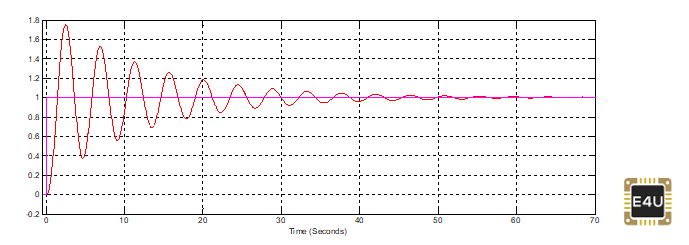Figure-9: Response of system shown in Figure-8, with K=5.8, Td=0.5

The transfer function of the PD controller is K+Tds or Td(s+K/Td); so we have added one zero at -K/Td. By controlling the value of ‘K’ or ‘Td’, the position of the ‘zero’ can be decided.

If ‘zero’ is very far away from the imaginary axis, its influence will decrease, if ‘zero’ is on the imaginary axis (or very close to the imaginary axis) it will also be not accepted (root locus generally starts from ‘poles’ & terminates at ‘zero’, Designer’s aim is generally such that root locus should not go towards the imaginary axis, due to this reason ‘zero’ very near to imaginary axis is also not acceptable, hence a moderate position of ‘zero’ should be kept)

Generally, it is said, PD controller improves transient performance and the PI controller improves the steady-state performance of a control system.

### Proportional plus Integral plus Derivative Controller (PID Controller)

A PID controller is generally used in industrial control applications to regulate temperature, flow, pressure, speed, and other process variables.

The transfer function of the PID Controller can be found as: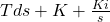or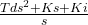It can be observed that one pole at origin is fixed, remaining parameters Td, K, and Ki decide the position of two zeros.

In this case, we can keep two complex zeros or two real zeros as per the requirement, hence PID controller can provide better tuning. In the olden days, the PI controller was one of the best choice of control engineers, because designing (tuning of parameters) of the PID controller was a little difficult, but nowadays, due to the development of software designing of PID controllers have become an easy task.

Against step input, For the values of K=5.8, Ki=0.2, and Td=0.5, Its time response, is shown in Fig-11. Compare Fig-11 with Fig-9 (We have taken values such that all the time response can be compared).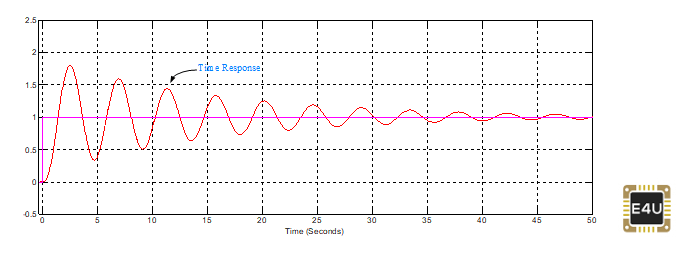Figure-11: Response of system shown in Figure-10, with K=5.8, Td=0.5, Ki=0.2

#### General Guidelines for Designing a PID Controller

When you are designing a PID controller for a given system, general guidelines to obtain the desired response are as follows:

1. Obtain the transient response of closed-loop transfer function and determine what needs to be improved.
2. Insert the proportional controller, Design the value of ‘K’ through Routh-Hurwitz or suitable software.
3. Add an integral part to reduce steady-state error.
4. Add the derivative part to increase damping (damping should be between 0.6-0.9). The derivative part will reduce overshoots & transient time.
5. Sisotool, available in MATLAB can also be used for proper tuning and to obtain a desired overall response.
6. Please note, above steps of tuning of parameters (designing of a control system) are general guidelines. There are no fixed steps for designing controllers.

### Fuzzy Logic controllers

Fuzzy Logic controllers (FLC) are used where systems are highly non-linear. Generally most of the physical systems/Electrical systems are highly non-linear. Due to this reason, Fuzzy Logic controllers are a good choice among researchers.

An accurate mathematical model is not needed in FLC. It works inputs based on past experiences, can handle non-linearities and can present disturbance insensitivity greater than most other non-linear controllers.

FLC is based on fuzzy sets, i.e. classes of objects in which the transition from membership to non-membership is smooth rather than abrupt.

In recent developments, FLC has outperformed other controllers in complex, nonlinear, or undefined systems for which good practical knowledge exists. Therefore, the boundaries of fuzzy sets can be vague and ambiguous, making them useful for approximation models.

The important step in the fuzzy controller synthesis procedure is to define the input and output variables based on previous experiences or practical knowledge.

This is done accordingly with the expected function of the controller. There are no general rules to select those variables, although typically the variables chosen are the states of the controlled system, their errors, error variation, and error accumulation.

Want To Learn Faster? 🎓
Get electrical articles delivered to your inbox every week.
No credit card required—it’s 100% free.About Dr Vipin Jain

Dr. Vipin Jain obtained a Bachelor of Engineering in 1992 from Nagpur University, a Masters of Technology in 2007, and a Ph.D. in 2017 from University of Delhi. He has been studying, working, and teaching in the electrical industry for over 25 years. He has been working as a faculty member in the Electrical Engineering Department of the Bharat Institute of Technology, Meerut (UP), India since December 2007.